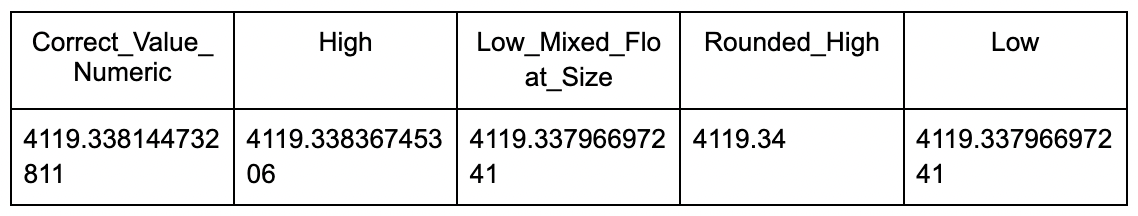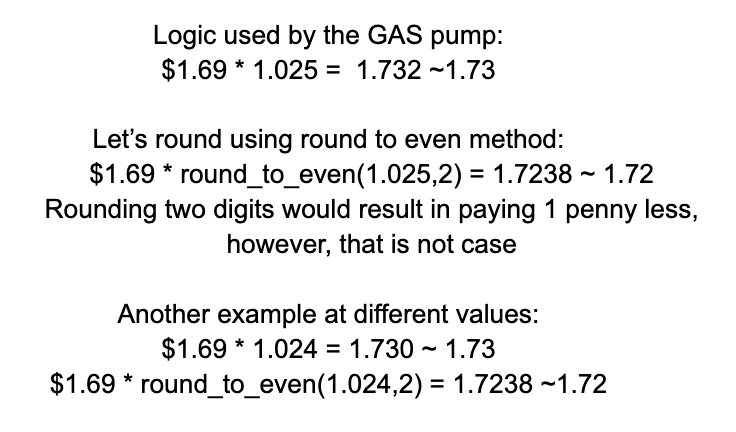##### PostgreSQL and Financial Calculations - Part OneA series on the use of data types to insure accurate financial calculations with your application.

Over my multi-decade career, I have often noticed the problematic use of real, floating, double, and fixed precision types to store and calculate financials. Most believe the application only needs two digits to the right of the decimal point for financial data. The use of only two digits assumes that many financial calculations do not need more than two decimal points; for example, the units of measure conversion or currency exchange. These transactions represent a significant monetary value that cannot be represented with only two digits of precision.

In this series we will go over common mistakes, what happens when you choose an incorrect data type, inconsistent precision scaling, conversion errors caused by frameworks and common calculation mistakes within PostgreSQL.

## Choosing the wrong data type:

One of the most common mistakes may be the most destructive. It is the use of the variable-precision data type (such as real, float and double precision) for financial calculations. Many view this as a non-issue because PostgreSQL stores and returns the value as it is received from the application. However, floating point types being 32 or 64 bit suffer from inexact math in an attempt to balance the precision of the values with the speed to calculate those values. This balance will introduce what appears to be a minor error, however, it will stack up to significant error. Below is an example showing what happens with different data types and variable precision when compared to the default use of numeric type.

### Consider 1:

PostgreSQL SQL

```SELECT
(1234.567 * 3.333333) + (1.234567 * 3.333333) as Correct_Value_Numeric,
(1234.567 * 3.333333)::float(25) + (1.234567 * 3.333333)::float(25) as High,
(1234.567 + 1.234567 )::float(24) * 3.333333::float(25) as Low_Mixed_Float_Size,
(1234.567 * 3.333333)::real + (1.234567 * 3.333333)::real as Rounded_High,
(1234.567 + 1.234567 )::real * 3.333333 as Low```With the above SQL query using casting, PostgreSQL uses floating type math operators demonstrating the errors that occur. Take note of the Low_Mixed_Float_Size column and odd math results when 32 and 64 bit floating point types. This kind of mixing of datatypes happens all the time in applications, which increases the value of the error.

Many will say this error is acceptable as it never impacts the 100th position and the rounded value is unchanged. This line of thinking is erroneous and ignores stackup. Consider what happens when buying a million widgets using the rounded price, or selling at the low price. Another counter argument is that only a few applications need this level of precision in financial calculations.

Let's look at a real world example of how precision and stackup are exploited. Gasoline pumps measure hydrocarbons to the 1,000th, but are priced in two digits of precision. Measuring the fuel to the 1,000th position to avoid rounding benefits the seller. Consider the following scenario where we compare the transaction using 3 digits of precision vs 2 digits:By keeping the fuel measurement at a higher precision and leaving the price at two digits, the seller is exploiting the stack up of selling a 1,000th of gallon instead of rounding it to the same precision as the price. This allows the seller to extract a tiny fraction of money over billions of measurements and transactions.

## The solution:

Be consistent with the data types. Don't mix real, double precision and numeric types (or the equivalent) in your favorite framework. Tiny errors will appear in casting of data type, either by truncation or rounding artifacts.

## Closing Thoughts:

The single biggest improvement in accuracy for calculations is increasing the level of precision of the numeric type, as this delays error creeping into the calculations.